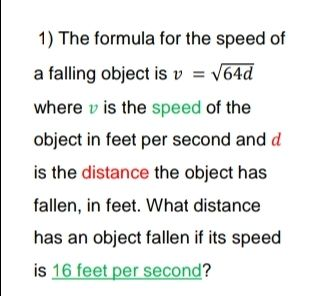### ¿Todavía tienes preguntas de matemáticas?

Pregunte a nuestros tutores expertos
Algebra
Pregunta1) The formula for the speed of a falling object is $$v = \sqrt { 64 d }$$

where $$v$$ is the speed of the object in feet per second and $$d$$ is the distance the object has fallen, in feet. What distance has an object fallen if its speed is $$16$$ feet per second?

$$16= \sqrt{64d}$$
$$16^{2} = 256= 64d$$
$$d= \frac{256}{64} = 4$$